## Identify and Write 2D or 3D Geometry Shape

Name
Date

1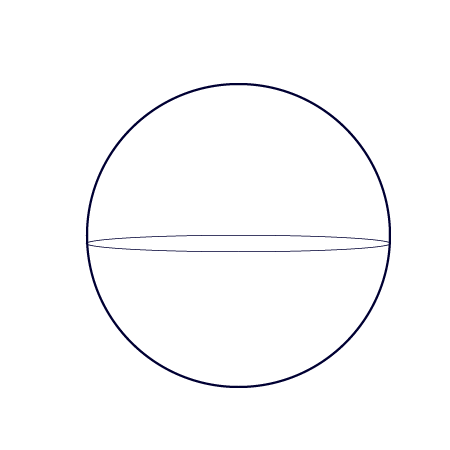2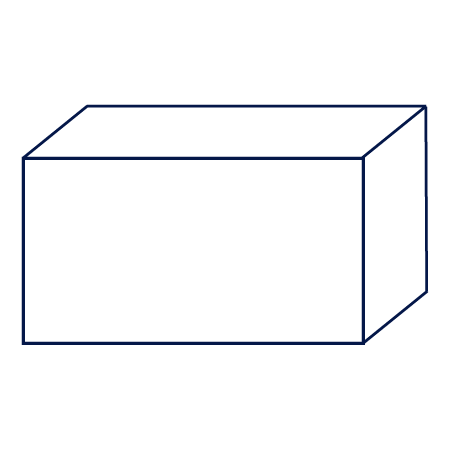3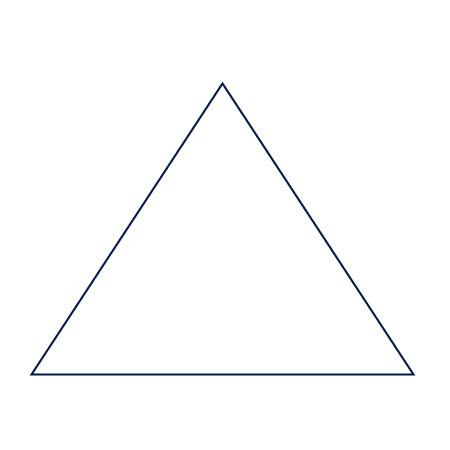4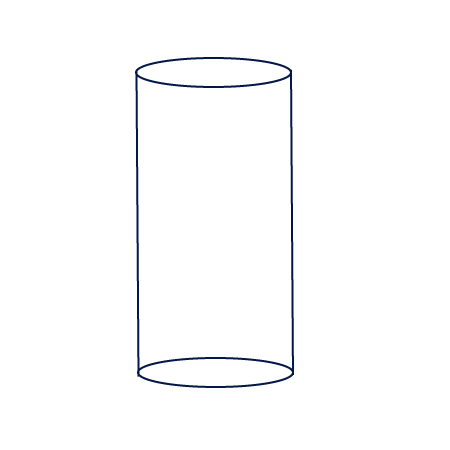5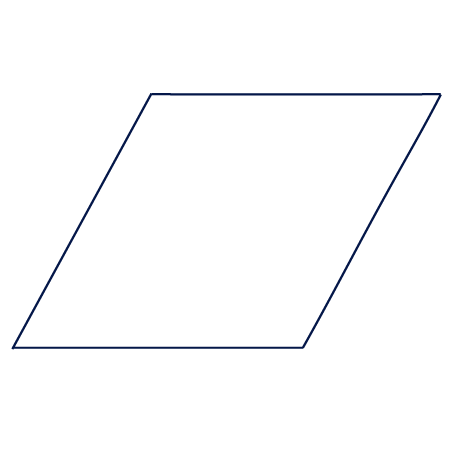6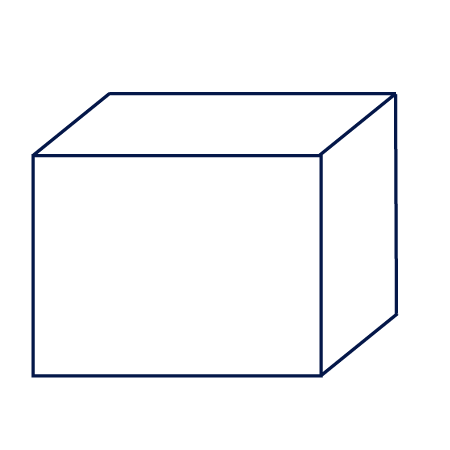7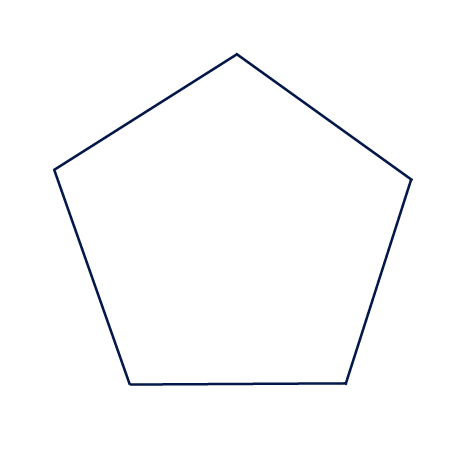8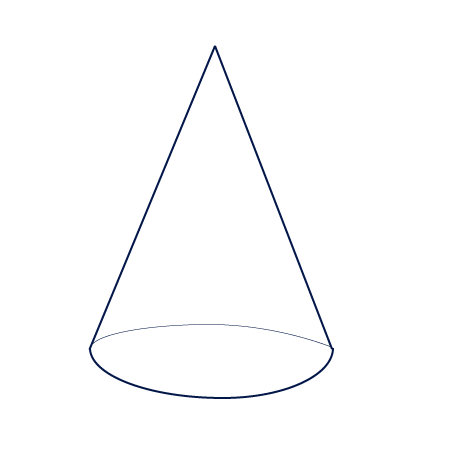9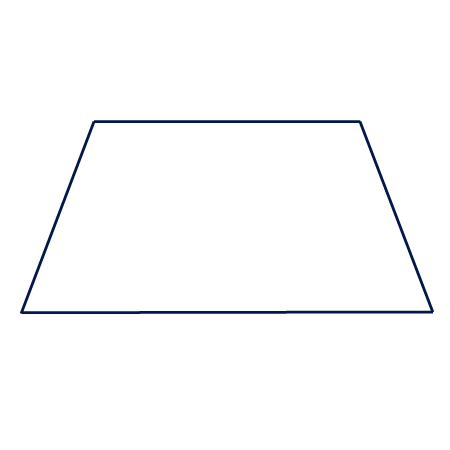10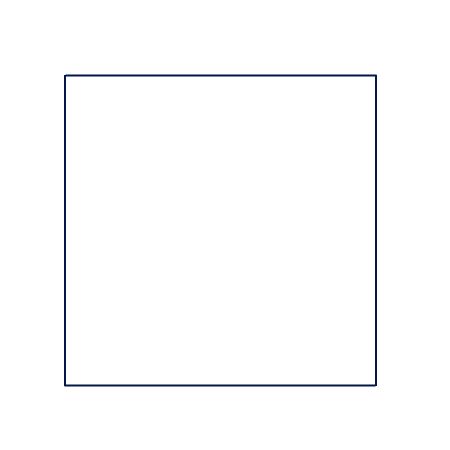13d Shape
23d Shape
32d Shape
43d Shape
52d Shape
63d Shape
72d Shape
83d Shape
92d Shape
102d Shape

# Identify 2D or 3D Geometry Shape Worksheet

The CCSS.MATH.K.G.A.3 worksheets with answers for kindergarten students to practice problems on Identify shapes as two dimensional or three-dimensional is available online for free in printable and downloadable (pdf & image) format. For more questions and answers on K.G.A.3 math activities, tap on generate new worksheet to learn, practice, test and verify math skills under the common core cluster (KGA) Identify and describe shapes (squares, circles, triangles, rectangles, hexagons, cubes, cones, cylinders, and spheres) in domain geometry.

How to Use this Worksheet

Objective:
To practice these math problems of KGA3 worksheets, students are required to identify shapes as two dimensional or three-dimensional. The main objective of these K.G.A.3 practice problems and answers is to make students to understand how to identify and describe shapes (squares, circles, triangles, rectangles, hexagons, cubes, cones, cylinders, and spheres). Users can directly refer the answer key to verify the work with steps of corresponding practice problems given in the K.G.A.3 activities under common core state standards for kindergarten mathematics.